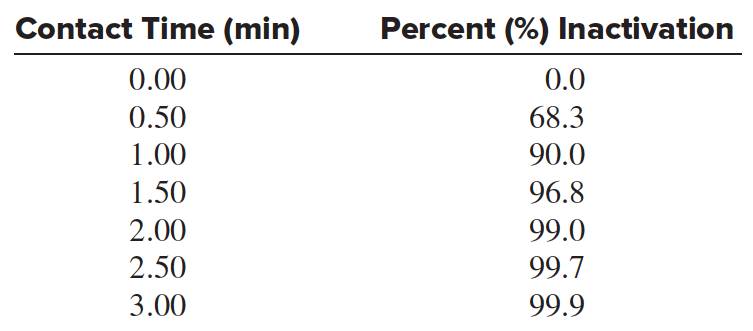# Problem: Chlorine is commonly used to disinfect drinking water, and inactivation of pathogens by chlorine follows first-order kinetics. The following data are for E. coli inactivation:Determine the first-order inactivation constant, k. [ Hint: % inactivation = 100 x (1 – [A] t/[A]0).]

###### FREE Expert Solution

We’re being asked to determine the first-order inactivation constant, k.

Recall that radioactive/nuclear decay of isotopes follows first-order kinetics, and the integrated rate law for first-order reactions is:

where:

[N]t = concentration at time, t
k = decay constant
t = time
[N]0 = initial concentration

We're given the equation for percent inactivation:

$\overline{)\mathbf{%}\mathbf{inactivation}\mathbf{=}\mathbf{100}\mathbf{×}\left(1-\frac{{\left[A\right]}_{t}}{{\left[A\right]}_{0}}\right)}$

Solving [A]t/[A]0 at 1 min:

88% (194 ratings)###### Problem Details

Chlorine is commonly used to disinfect drinking water, and inactivation of pathogens by chlorine follows first-order kinetics. The following data are for E. coli inactivation:Determine the first-order inactivation constant, k. [ Hint: % inactivation = 100 x (1 – [A] t/[A]0).]

Frequently Asked Questions

What scientific concept do you need to know in order to solve this problem?

Our tutors have indicated that to solve this problem you will need to apply the Integrated Rate Law concept. You can view video lessons to learn Integrated Rate Law. Or if you need more Integrated Rate Law practice, you can also practice Integrated Rate Law practice problems.

What textbook is this problem found in?

Our data indicates that this problem or a close variation was asked in Chemistry: The Molecular Nature of Matter and Change - Silberberg 8th Edition. You can also practice Chemistry: The Molecular Nature of Matter and Change - Silberberg 8th Edition practice problems.